Example Questions

Example Question #1 : Geometry

If a rectangle possesses a width of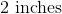and has a perimeter of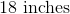, then what is the length?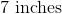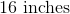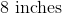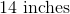Explanation:

In order to solve this problem, we need to recall the formula for perimeter of a rectangle: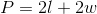We can substitute in our known values and solve for our unknown variable (i.e. length):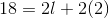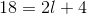We want to isolate theto one side of the equation. In order to do this, we will first subtractfrom both sides of the equation.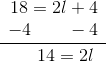Next, we can divide each side by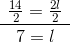The length of the rectangle isExample Question #5 : 7th Grade Math

The figure represents a set of supplementary angles, solve for.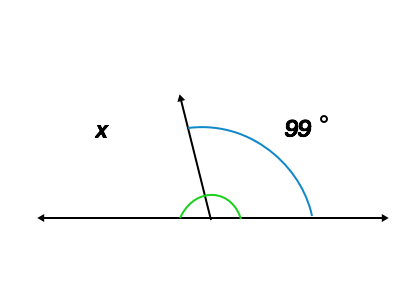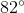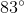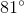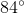Explanation:

Supplementary angles are defined as two angles that when added together equalFrom the question, we know that the two angles are supplementary, and thus equal, so we can set up the following equation: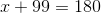Next we can solve for: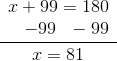Example Question #1 : 7th Grade Math

What is the area of the circle provided?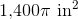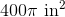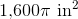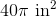Explanation:

In order to solve this problem, we need to recall the formula for the area of a circle: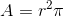The circle in this question provides us with the diameter, so we first have to solve for the radius. Remember, the radius is half the diameter: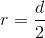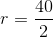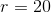Now that we have the radius we can use the formula to solve: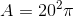Solve: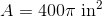Example Question #7 : 7th Grade Math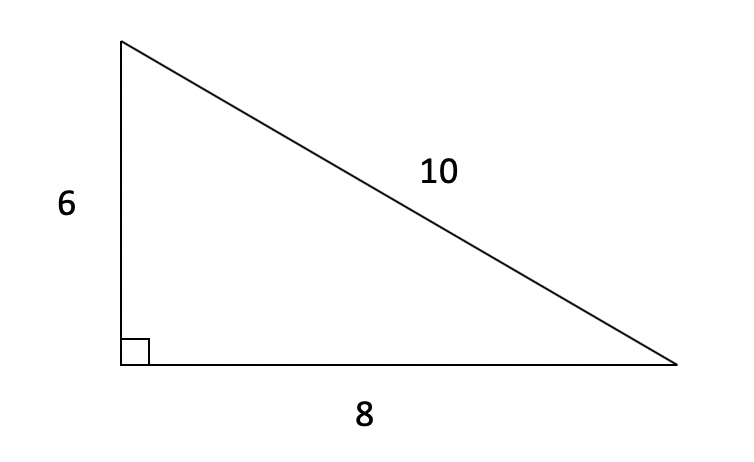What is the area of the triangle pictured above?

40

30

24

12

60

The area of a triangle is calculated using the formula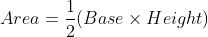.  Importantly, the height is a perpendicular line between the base and the opposite point.  In a right triangle like this one, you're in luck: the triangle as drawn already has that perpendicular line as one of the two sides.  So here we will calculate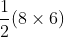.  That gives us an answer of 24.